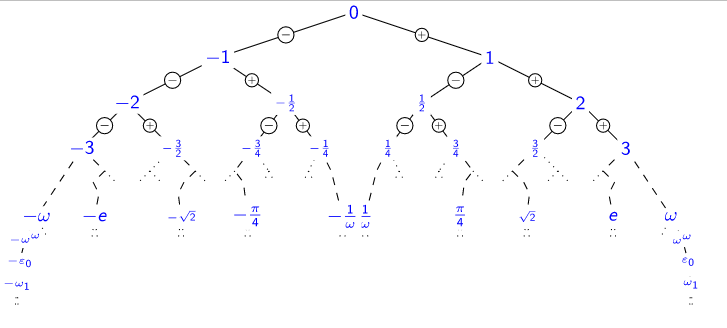# Model theory of analytic functionsThe function that measures, for instance, the position of a pendulum as time passes, is under ideal conditions (such as no friction) very smooth, without sudden jumps, and it belongs in fact to a class of very smooth functions called "analytic". Analytic functions emerge naturally in a wide variety of contexts, from abstract mathematics to models of real-world phenomena.

Such functions are well behaved locally; for functions of time, as in the example of the pendulum, it means well behaved in sufficiently short time intervals. But wildly different behaviours can emerge when considered globally, i.e. after longer and longer periods of time, such as exponential growth (e.g. the size of a debt accumulating interest over time), exponential decay (the radioactivity of radioactive waste), or oscillation (as per a pendulum without friction).

Model theory, a branch of mathematical logic, provides a sharp divide between analytic functions that oscillate and functions that do not, and a further distinction between oscillating functions. A real analytic function lies in an "o-minimal structure" if every set of real numbers defined by a first-order formula involving the function is made of finitely many points and intervals, thus no oscillation may appear. On the other hand, a complex analytic function, which must oscillate as soon as it is transcendental, lies in a "quasi-minimal structure" if every set of complex numbers defined by a first order formula is either countable, or its complement is countable.

The aim of this fellowship is to shed light on the o-minimality and quasi-minimality of classes of functions of interest in mathematics. The o-minimality of real exponentiation, known since the 1990s, had and still has a major impact across mathematics, from number theory to analysis. It is still an open problem whether there are o-minimal functions that grow much faster than exponentially (called transexponential), which would have implications on dynamical systems, such as around Hilbert's 16th problem on polynomial vector fields. The quasi-minimality of complex exponentiation is still one of the big problems in model theory, 25 years after it was first conjectured, and a positive answer is likely to have far-reaching consequences as did the o-minimality of real exponentiation.

I will use Conway's surreal numbers (an extension of real numbers with infinite and infinitesimal numbers, encompassing both reals and ordinals) to investigate Hardy fields, which are classes of real non-oscillating functions, and transseries, which are formal asymptotic expansions meant to represent them; and in particular tackle the problem of the existence of o-minimal transexponential functions. I will do so by strengthening the recently discovered connections between surreal numbers, transseries and Hardy fields; creating and analysing the model theory of transexponential functions on surreal numbers; introducing a framework that ties together functions on surreal numbers, non-oscillating functions and o-minimality.

Furthermore, I will investigate the quasi-minimality of complex exponentiation and analogous structures. I will do so by proving instances of exponential-algebraic closure, which predicts when systems of polynomial-exponential equations should have complex solutions, and extending the results to other exponential functions arising from abelian varieties and their extensions, paving the way for a universal quasi-minimal structure containing all the exponential functions of commutative algebraic groups.

### Project website

https://gow.epsrc.ukri.org/NGBOViewGrant.aspx?GrantRef=EP/T018461/1